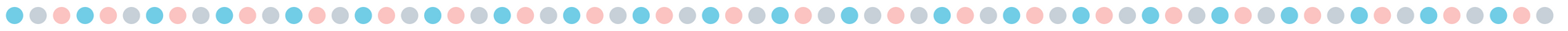## Hands-On Counting Practice with Hole Punch Counting

Let’s make counting fun with a hands-on math activity! All you need is a hole punch and print the free Hole Punch Activity PDF.

These hole punch counting activities help kids practice counting and allow children to strengthen hand muscles and motor skills by using a hole punch.

This counting activity is perfect for preschool, pre-k, and kindergarten-age children.

You can make the activity extra special by using fun-shaped hole punches. Amazon has a three-pack that contains a heart, circle, and star.

###### Follow:

Math Mats help students visualize the math as they are learning and growing their math skills. They also give students the opportunity to be hands-on while learning.
Ways to Use the Math Mats:
• With a dry erase marker only: (The fine tip dry erase markers work better)
• You announce two numbers and the students write the numbers in each turkey, then the students complete the addition or subtraction sentence. or
• You announce two numbers and the students make dots in each turkey to represent the numbers, then the students complete the addition or subtraction sentence.
• With a dry erase marker and erasers:
• You can announce two numbers and the students put the number of erasers in each turkey, then the students complete the addition or subtraction sentence
• With a dry erase marker and dice:
• Students roll their dice, then place each die in the turkey, then the students complete the addition or subtraction sentence.
• You could use dice with 4, 6, 8,  10, 12, or 20 sided dice to differentiate your instruction.
Supplies:

## St. Patrick's Day Ten Frame with Teen Number Practice

Ten frames help kids develop number sense. It teaches them to subitize and is a precursor to addition.  Ten frames provide students with a visual of numbers and give children experiences working with numbers. With the ten frame strategy students aren’t memorizing numbers or counting without meaning. Ten frames help students under the value of numbers.

You can print and have students write directly on the sheet or you can print and place it in a dry-erase pocket so you can print once and use it many times.
More St. Patrick’s Day Learning Activities:

## St Patrick's Day Single-Digit Addition Practice

Are you looking for some quick one-digit addition practice? This free resource has three different versions of the St. Patrick’s Day single-digit addition.

###### Follow:

St. Patrick’s day is just around the corner and these free printable counting sheets are a great way to add some St. Patrick’s Day flair to math practice.
You can print and have students write directly on the sheet or you can print and place it in a dry-erase pocket so you can print once and use it many times.
You can also pair this with small erasers. Students place an eraser on each leaf and count as they place them. Then the student writes the number on the line and then counts as they collect the erasers. This way it is hands-on and students are counting more.

## Valentine's Day Math Mats (Free printable)

Ways to Use the Math Mats:
• With a dry erase marker only: (The fine tip dry erase markers work better)
• You announce two numbers and the students write the numbers in each turkey, then the students complete the addition or subtraction sentence. or
• You announce two numbers and the students make dots in each turkey to represent the numbers, then the students complete the addition or subtraction sentence.
• With a dry erase marker and erasers:
• You can announce two numbers and the students put the number of erasers in each turkey, then the students complete the addition or subtraction sentence
• With a dry erase marker and dice:
• Students roll their dice, then place each die in the turkey, then the students complete the addition or subtraction sentence.
• You could use dice with 4, 6, 8,  10, 12, or 20 sided dice to differentiate your instruction.
Supplies: